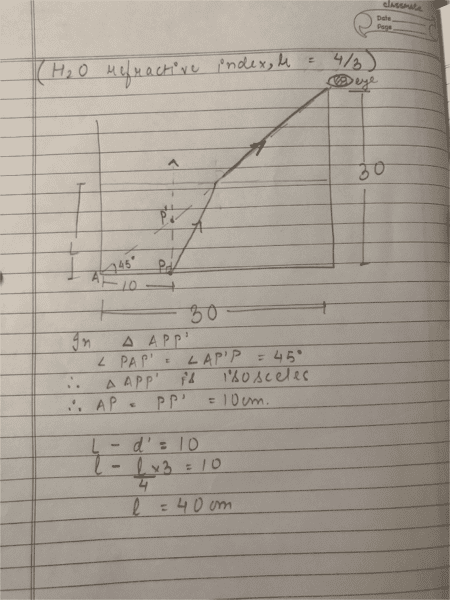# Apparent depth

I1. Homework Statement
A cylindrical vessel whose diameter and height both are equal to 30 cm is placed on a horizontal surface and a small particle p is placed in it at a distance of 5 cm from the centre. An eye is placed at a position such that the edge of the bottom is in the plane of drawing. Upto what minimum height should water be filled in the vessel to make particle p visible?

## Homework Equations

Apparent depth (d’) = real depth (d) / refractive index

## The Attempt at a Solution

My answer is 40 cm which is wrong.[/B]

#### Attachments

Last edited by a moderator:

haruspex
Homework Helper
Gold Member
Apparent depth (d’) = real depth (d) / refractive index
That formula is for looking straight down, i.e. the line of vision is normal to the surface,

That formula is for looking straight down, i.e. the line of vision is normal to the surface,

But why do the rays bend if it’s for looking straight down? Because their Sin 0 *4/3 = sin r
And r would have to be 0 so the rays wouldn’t bent then why does it appear to be raised?

sorry. I mean sin i *4/3 = sin 0
Sin i = 0
Could you please explain why that equation is used for when an object is viewed from straight above.
I'm having a problem with that equation in pther questions also.

haruspex
Homework Helper
Gold Member
But why do the rays bend if it’s for looking straight down?
Your pupil has diameter. Consider, from a point object, one ray to each side of the iris. These are not quite vertical, so as they emerge will be bent just a little away from each other. Project the emerging rays back down to the point where they intersect. This will be where the object appears to be.

The calculation of apparent depth assumes these angles are small. In the problem you are given the angles are much larger.

These are not quite vertical, so as they emerge will be bent just a little away from each other.

But the lens in our eyes is convex and it converges the rays. So why will the rays bend away from each other?

Sorry I didn't get what you said earlier. The rays bend away from each otherat the surface of the liquid. Thanks for helping me out.

How do you get the answer for this question?

https://ibb.co/k0zFv8

haruspex
Homework Helper
Gold Member
Sorry I didn't get what you said earlier. The rays bend away from each otherat the surface of the liquid. Thanks for helping me out.

How do you get the answer for this question?

https://ibb.co/k0zFv8
Draw a ray from the object that is a small angle θ above the horizontal. (You can draw it large for legibility but bear in mind that is in principle small.)
Show how it deviates at each interface before reaching the eye.
We can take the horizontal line as a second ray, not deviating.
Project back the final section of the first until it meets the horizontal one. That is where the object will appear to be.
Now use some geometry to relate the apparent distance to the other distances and the angles.